# 带你走进 WebGL 的随机美学## 概述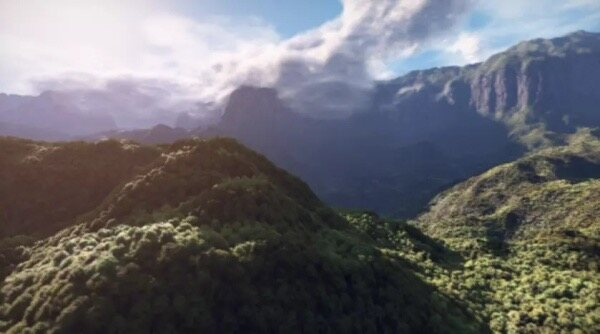Noise 构造地形、体积云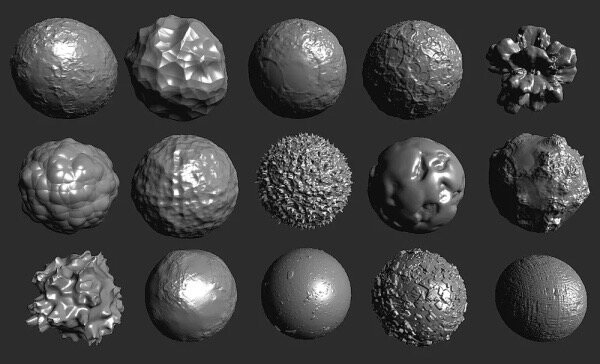## 基础噪声算法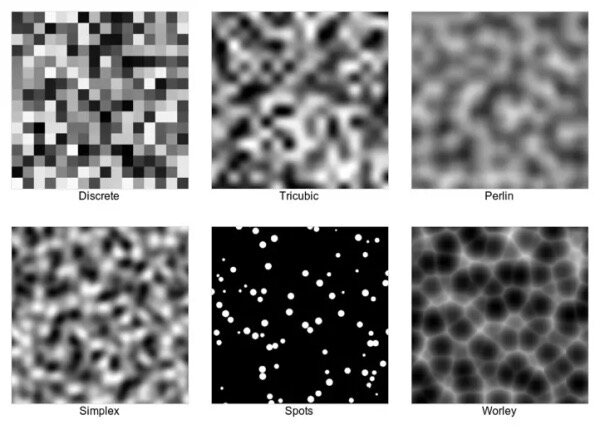// noise fragment shadervarying vec2 uv;float noise(vec2 p) {  // TODO}void main() {    float n = noise(uv);  // 通过噪声函数计算片元坐标对应噪声值    gl_FragColor = vec4(n, n, n, 1.0);}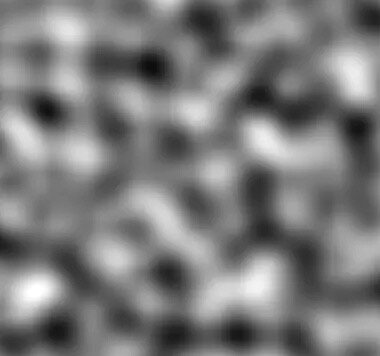Perlin Noise 为 Perlin 提出的噪声算法

#### 算法步骤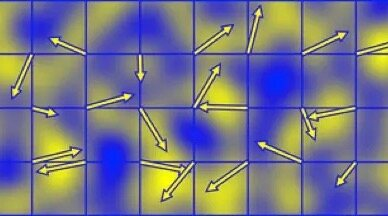Perlin Noise 随机向量代表梯度

#define SCALE 4. // 将平面分为 4 × 4 个正方形网格float noise(vec2 p) {  p *= SCALE;  // TODO}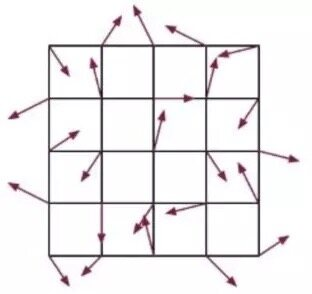// 输入网格顶点位置，输出随机向量vec2 random(vec2 p){	return  -1.0 + 2.0 * fract(		sin(			vec2(				dot(p, vec2(127.1,311.7)),				dot(p, vec2(269.5,183.3))			)		) * 43758.5453	);}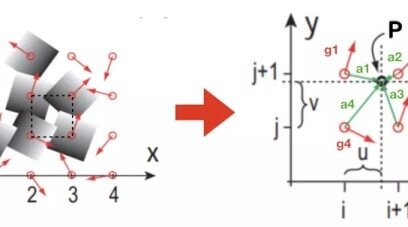float noise_perlin (vec2 p) {    vec2 i = floor(p); // 获取当前网格索引 i    vec2 f = fract(p); // 获取当前片元在网格内的相对位置    // 计算梯度贡献值    float a = dot(random(i),f); // 梯度向量与距离向量点积运算    float b = dot(random(i + vec2(1., 0.)),f - vec2(1., 0.));    float c = dot(random(i + vec2(0., 1.)),f - vec2(0., 1.));    float d = dot(random(i + vec2(1., 1.)),f - vec2(1., 1.));    // 平滑插值    vec2 u = smoothstep(0.,1.,f);    // 叠加四个梯度贡献值    return mix(mix(a,b,u.x),mix(c,d,u.x),u.y);}

### 细胞噪声 (Celluar Noise)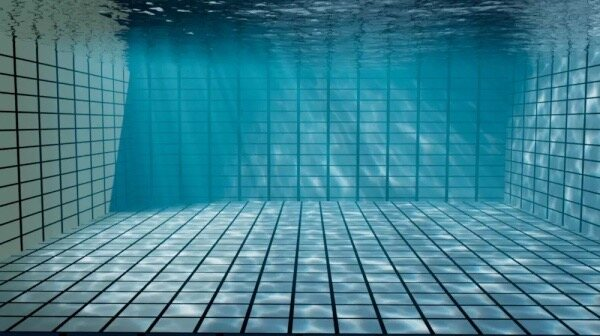Celluar Noise 生成的噪声图由很多个“晶胞”组成，每个晶胞向外扩张，晶胞之间相互抑制。这类噪声可以模拟细胞形态、皮革纹理等。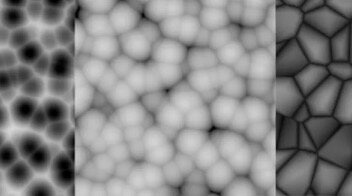noise

#### 算法步骤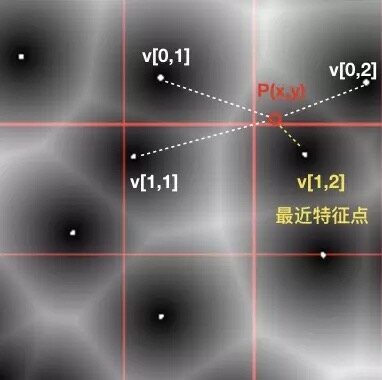// 输入网格索引，输出网格特征点坐标vec2 random(vec2 st){	return  fract(		sin(			vec2(				dot(st, vec2(127.1,311.7)),				dot(st, vec2(269.5,183.3))			)		) * 43758.5453	);}

float noise(vec2 p) {    vec2 i = floor(p); // 获取当前网格索引 i    vec2 f = fract(p); // 获取当前片元在网格内的相对位置    float F1 = 1.;    // 遍历当前像素点相邻的 9 个网格特征点    for (int j = -1; j <= 1; j++) {        for (int k = -1; k <= 1; k++) {            vec2 neighbor = vec2(float(j), float(k));            vec2 point = random(i + neighbor);            float d = length(point + neighbor - f);            F1 = min(F1,d);        }    }    return F1;}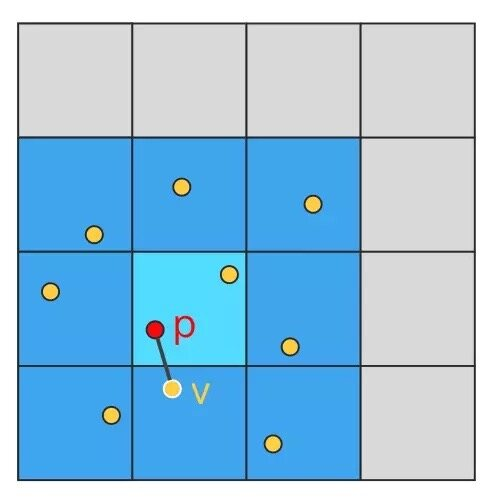## 噪声算法组合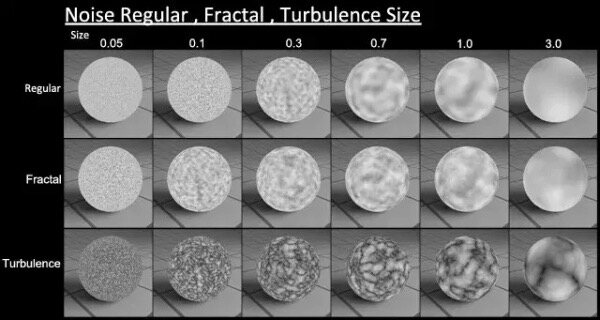### 分形布朗运动（Fractal Brownian Motion）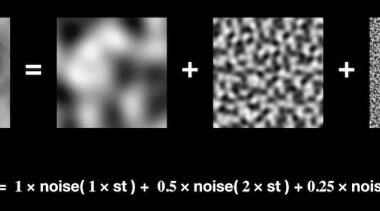• 公式：fbm = noise(st) + 0.5 * noise(2*st) + 0.25 * noise(4*st)

// fragment shader 片元着色器#define OCTAVE_NUM 5// 叠加 5 次的分形噪声float fbm_noise(vec2 p){    float f = 0.0;    p = p * 4.0;    float a = 1.;    for (int i = 0; i < OCTAVE_NUM; i++)    {        f += a * noise(p);        p = 4.0 * p;        a /= 4.;    }    return f;}

### 湍流（Turbulence）

• 公式：fbm = |noise(st)| + 0.5 * |noise(2*st)| + 0.25 * |noise(4*st)|

// 湍流分形噪声float fbm_abs_noise(vec2 p){    ...    for (int i = 0; i < OCTAVE_NUM; i++)    {        f += a * abs(noise(p)); // 对噪声函数取绝对值        ...    }    return f;}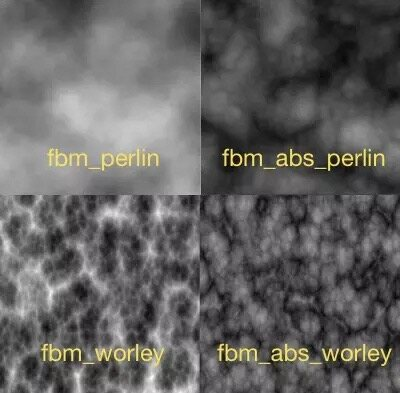Perlin Noise 与 Worley Noise 的 2D 分形

### 翘曲域（Domain Wrapping）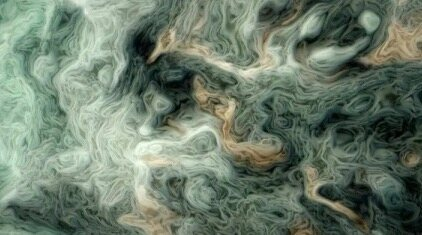• 公式：f(p) = fbm( p + fbm( p + fbm( p ) ) )

float domain_wraping( vec2 p ){    vec2 q = vec2( fbm(p), fbm(p) );     vec2 r = vec2( fbm(p + q), fbm(p + q) );     return fbm( st + r );}

### 动态纹理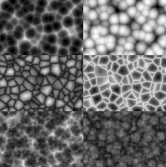2D + Time 动态噪声

// noise fragment shader#define SPEED 20.varying vec2 uv;uniform float u_time;float noise(vec3 p) {  // TODO}void main() {    float n = noise(uv, u_time *  SPEED);  // 传入片元坐标与时间    gl_FragColor = vec4(n, n, n, 1.0);}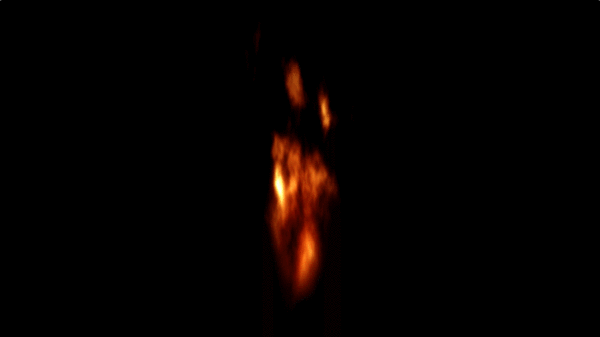Noise 制作火焰

## 噪声贴图应用

### Color Mapping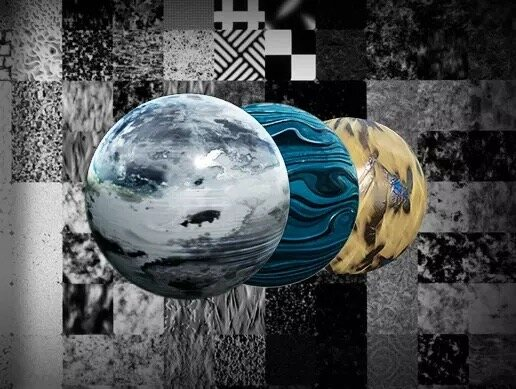### Height MappingFbm Perlin Noise→heightmap→山脉

### Normal Mapping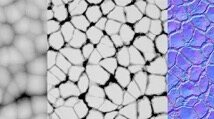Worley Noise→Normalmap→地表细节

## 噪声贴图实践

1. 读取一张静态 noise 图片的噪声值；

2. 加载 noise 程序，切换着色器中运行它；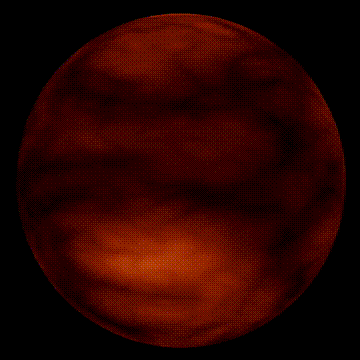demo 预览： https://yonechen.github.io/webgl-noise-examples/web/index.html

class Web3d {    constructor() { ... } // 创建场景、相机、渲染器    // 渲染前初始化钩子    start() {        this.addLight(); // 添加灯光        this.addBall(); // 添加一个球体    }    addBall() {        const { scene } = this;        this.initNoise();        const geometry = new THREE.SphereBufferGeometry(50, 32, 32); // 创建一个半径为 50 的球体        // 创建材质        const material = new THREE.MeshPhongMaterial( {            shininess: 5,            map: this.colorMap.texture // 将噪声纹理作为球体材质的 colorMap        } );        const ball = new THREE.Mesh( geometry, material );        ball.rotation.set(0,-Math.PI,0);        scene.add(ball);    }    // 动态渲染更新钩子    update() { }}

const ColorMapShader = {    uniforms: {        "scale": { value: new THREE.Vector2( 1, 1 ) },        "offset": { value: new THREE.Vector2( 0, 0 ) },        "time": { value: 1.0 },    },    vertexShader:         varying vec2 vUv;        uniform vec2 scale;        uniform vec2 offset;{1}        void main( void ) {            vUv = uv * scale + offset;            gl_Position = projectionMatrix * modelViewMatrix * vec4( position, 1.0 );        }    ,    fragmentShader:         varying vec2 vUv;        uniform float time;        vec3 random_perlin( vec3 p ) {            p = vec3(                    dot(p,vec3(127.1,311.7,69.5)),                    dot(p,vec3(269.5,183.3,132.7)),                     dot(p,vec3(247.3,108.5,96.5))                     );            return -1.0 + 2.0*fract(sin(p)*43758.5453123);        }        float noise_perlin (vec3 p) {            vec3 i = floor(p);            vec3 s = fract(p);{1}            // 3D 网格有 8 个顶点            float a = dot(random_perlin(i),s);            float b = dot(random_perlin(i + vec3(1, 0, 0)),s - vec3(1, 0, 0));            float c = dot(random_perlin(i + vec3(0, 1, 0)),s - vec3(0, 1, 0));            float d = dot(random_perlin(i + vec3(0, 0, 1)),s - vec3(0, 0, 1));            float e = dot(random_perlin(i + vec3(1, 1, 0)),s - vec3(1, 1, 0));            float f = dot(random_perlin(i + vec3(1, 0, 1)),s - vec3(1, 0, 1));            float g = dot(random_perlin(i + vec3(0, 1, 1)),s - vec3(0, 1, 1));            float h = dot(random_perlin(i + vec3(1, 1, 1)),s - vec3(1, 1, 1));{1}            // Smooth Interpolation            vec3 u = smoothstep(0.,1.,s);{1}            // 根据八个顶点进行插值            return mix(mix(mix( a, b, u.x),                        mix( c, e, u.x), u.y),                    mix(mix( d, f, u.x),                        mix( g, h, u.x), u.y), u.z);        }        float noise_turbulence(vec3 p)        {            float f = 0.0;            float a = 1.;            p = 4.0 * p;            for (int i = 0; i < 5; i++) {                f += a * abs(noise_perlin(p));                p = 2.0 * p;                a /= 2.;            }            return f;        }        void main( void ) {            float c1 = noise_turbulence(vec3(vUv, time/10.0));            vec3 color = vec3(1.5*c1, 1.5*c1*c1*c1, c1*c1*c1*c1*c1*c1);            gl_FragColor = vec4( color, 1.0 );        }    };

OK，现在让 WebGL 去加载这段程序，并告诉它这段代码是要作为球体的纹理贴图的：

    initNoise() {        const { scene, renderer } = this;        // 创建一个噪声平面，作为运行噪声 shader 的载体。        const plane = new THREE.PlaneBufferGeometry( window.innerWidth, window.innerHeight );        const colorMapMaterial = new THREE.ShaderMaterial( {            ...ColorMapShader, // 将噪声着色器代码传入 ShaderMaterial            uniforms: {                ...ColorMapShader.uniforms,                scale: { value: new THREE.Vector2( 1, 1 ) }            },            lights: false        } );        const noise = new THREE.Mesh( plane, colorMapMaterial );        scene.add( noise );        // 创建噪声纹理的渲染对象 framebuffer。        const colorMap = new THREE.WebGLRenderTarget( 512, 512 );        colorMap.texture.generateMipmaps = false;        colorMap.texture.wrapS = colorMap.texture.wrapT = THREE.RepeatWrapping;        this.noise = noise;        this.colorMap = colorMap;        this.uniformsNoise = colorMapMaterial.uniforms;        // 创建一个正交相机，对准噪声平面。        this.cameraOrtho = new THREE.OrthographicCamera( window.innerWidth / - 2, window.innerWidth / 2, window.innerHeight / 2, window.innerHeight / - 2, - 10000, 10000 );        this._renderNoise();    }

    _renderNoise() {        const { scene, noise, colorMap, renderer, cameraOrtho } = this;        noise.visible = true;        renderer.setRenderTarget( colorMap );        renderer.clear();        renderer.render( scene, cameraOrtho );        noise.visible = false;    }    update(delta) {        this.uniformsNoise[ 'time' ].value += delta; // 更新 noise 的时间，生成动态纹理        this._renderNoise();    }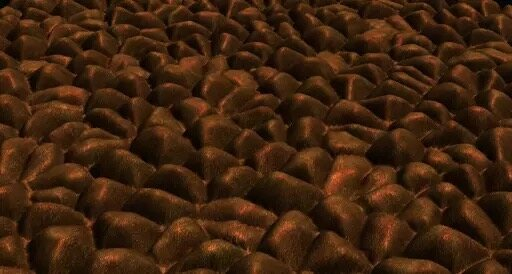Worley Noise 构造地形